# SECTION 2 2 Linear Equations Graphing Linear Equations

• Slides: 26SECTION 2. 2 Linear Equations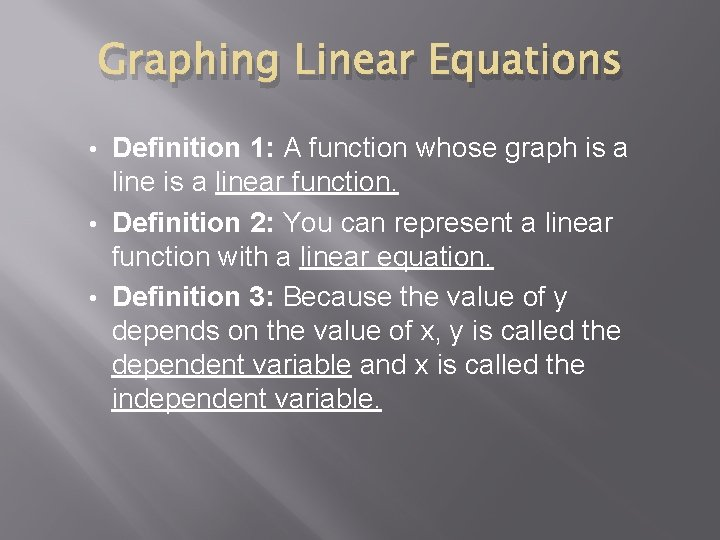Graphing Linear Equations Definition 1: A function whose graph is a linear function. • Definition 2: You can represent a linear function with a linear equation. • Definition 3: Because the value of y depends on the value of x, y is called the dependent variable and x is called the independent variable. •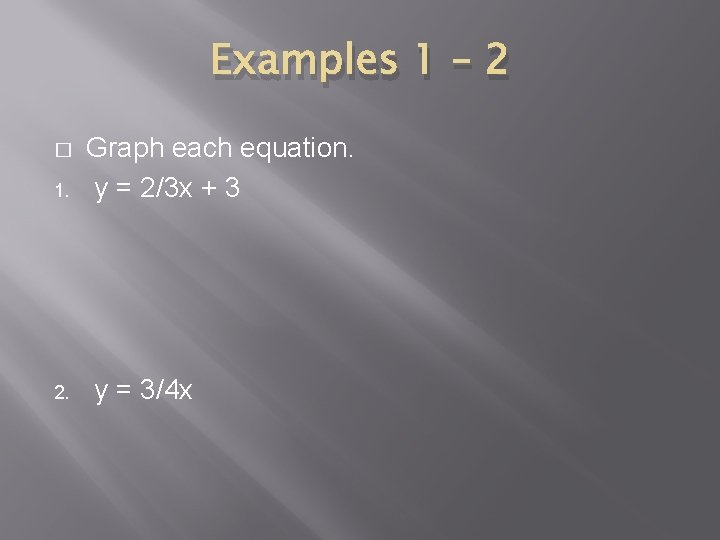Examples 1 – 2 � 1. 2. Graph each equation. y = 2/3 x + 3 y = 3/4 x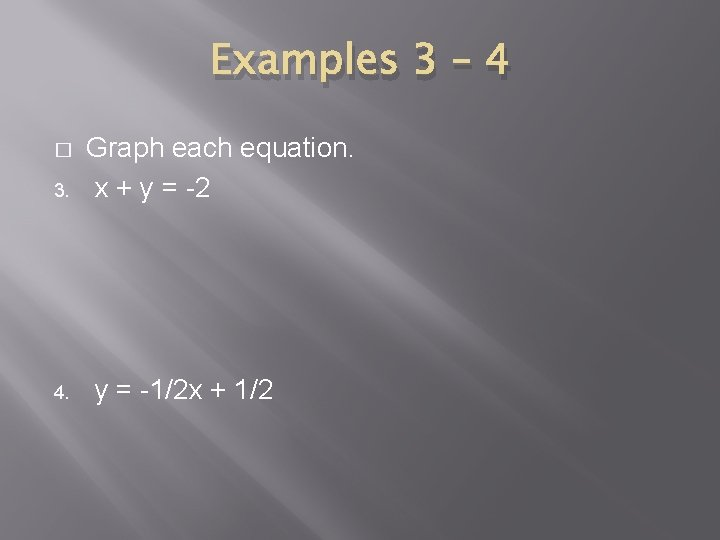Examples 3 – 4 � 3. 4. Graph each equation. x + y = -2 y = -1/2 x + 1/2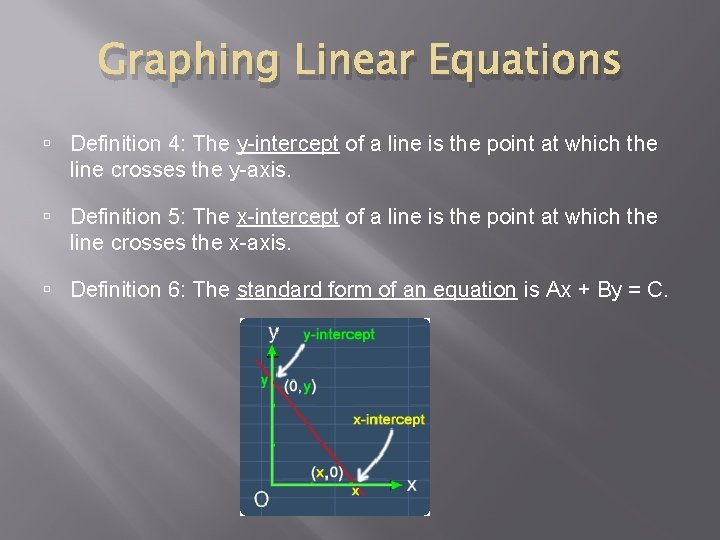Graphing Linear Equations Definition 4: The y-intercept of a line is the point at which the line crosses the y-axis. Definition 5: The x-intercept of a line is the point at which the line crosses the x-axis. Definition 6: The standard form of an equation is Ax + By = C.Example 5 � Transportation: The equation 3 x + 2 y = 120 models the number of passengers who sit in a train car, where x is the number of adults and y is the number of children. Graph the equation. Explain what the x- and y-intercepts represent. Describe the domain and the range.Example 5 Continued � Suppose the train system buys new train cars with molded plastic seats. The model changes to x + y = 40. Graph the equation and interpret the x- and y-intercepts.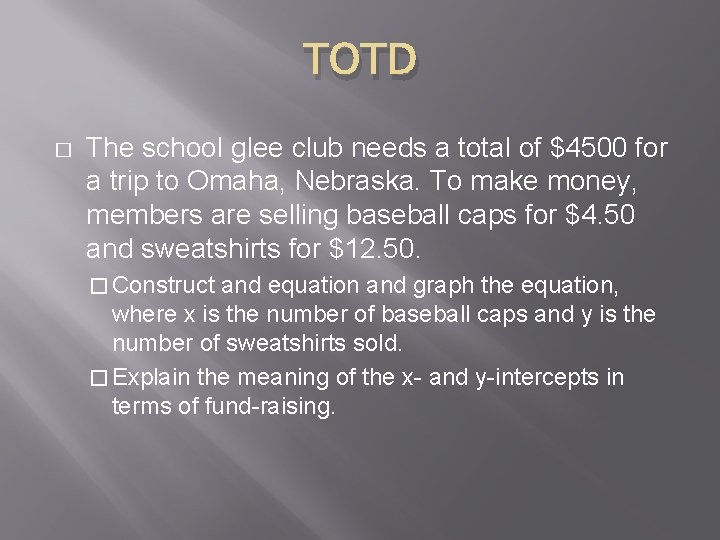TOTD � The school glee club needs a total of \$4500 for a trip to Omaha, Nebraska. To make money, members are selling baseball caps for \$4. 50 and sweatshirts for \$12. 50. � Construct and equation and graph the equation, where x is the number of baseball caps and y is the number of sweatshirts sold. � Explain the meaning of the x- and y-intercepts in terms of fund-raising.Slope � Definition 7: The slope of a nonvertical line is the ratio of the vertical change to a corresponding horizontal change.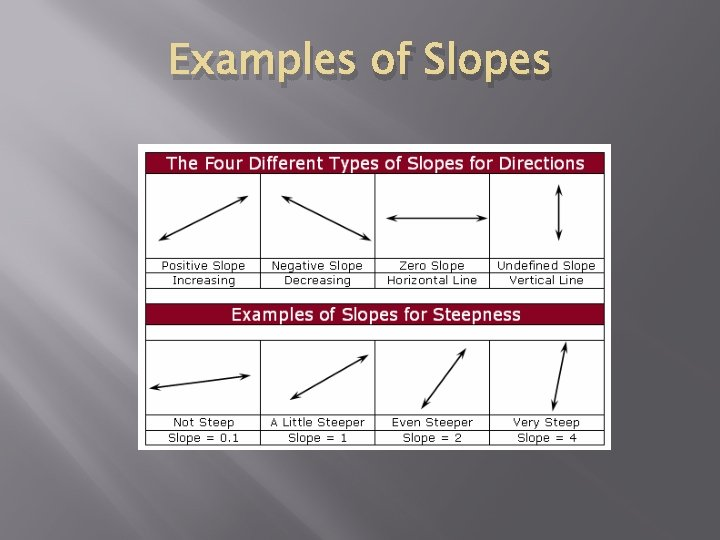Examples of Slopes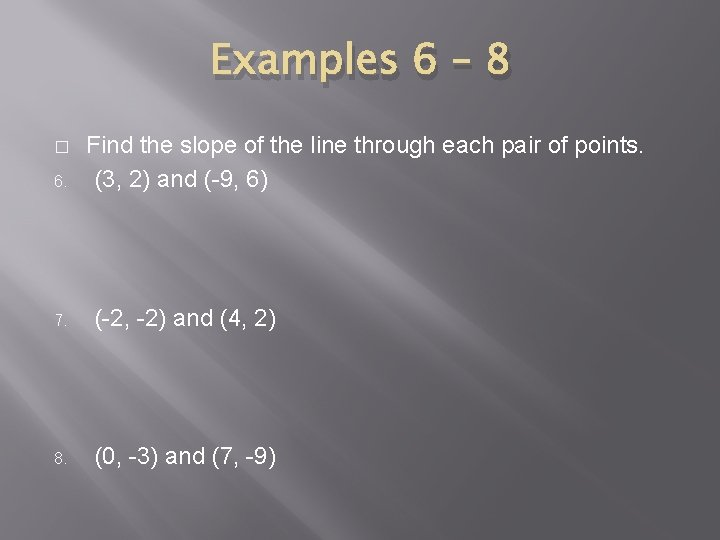Examples 6 – 8 � 6. Find the slope of the line through each pair of points. (3, 2) and (-9, 6) 7. (-2, -2) and (4, 2) 8. (0, -3) and (7, -9)Writing Equations of Lines � Definition 8: When you know the slope and a point on a line, you can use the point-slope form to write the equation of a line.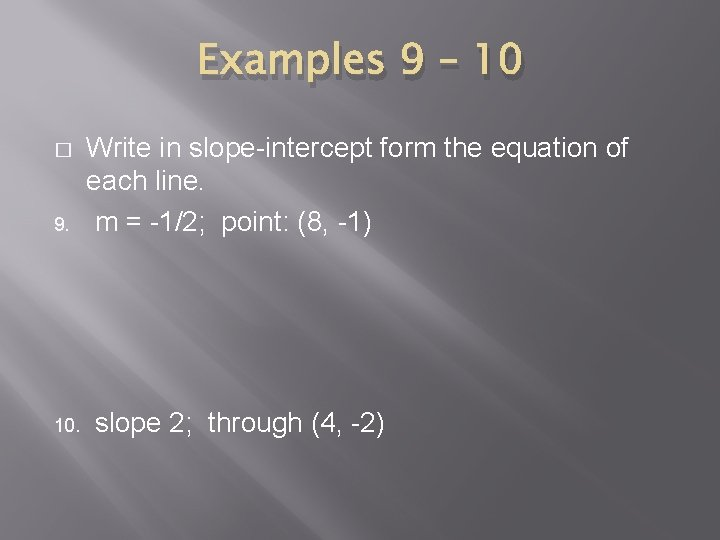Examples 9 – 10 � 9. 10. Write in slope-intercept form the equation of each line. m = -1/2; point: (8, -1) slope 2; through (4, -2)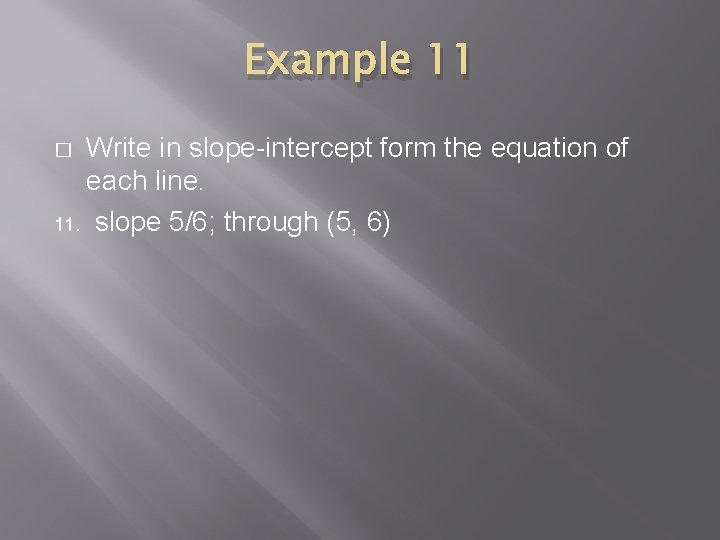Example 11 Write in slope-intercept form the equation of each line. 11. slope 5/6; through (5, 6) �TOTD � Find the slope of the line through each pair of points. � (1, � 6) and (8, -1) Write the equation of the line. Graph. � slope = 3; (1, 5)Examples 12 – 13 � 12. 13. Write in slope-intercept form the equation of the line through each pair of points. (1, 5) and (4, -1) (5, 0) and (-3, 2)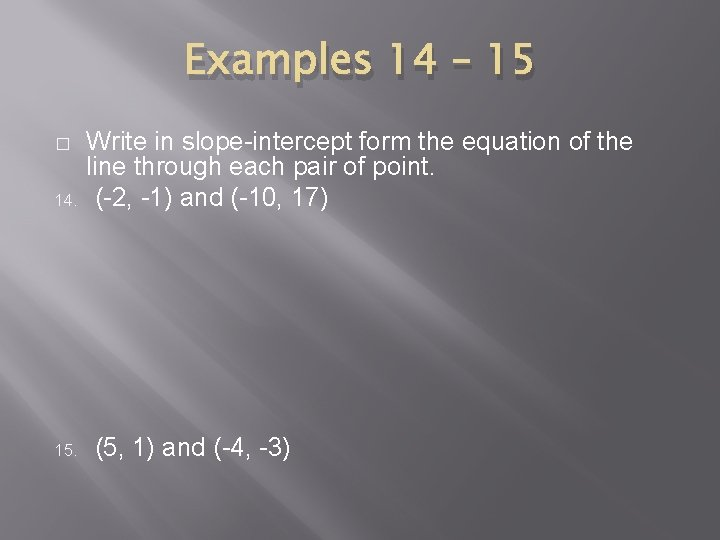Examples 14 – 15 � 14. 15. Write in slope-intercept form the equation of the line through each pair of point. (-2, -1) and (-10, 17) (5, 1) and (-4, -3)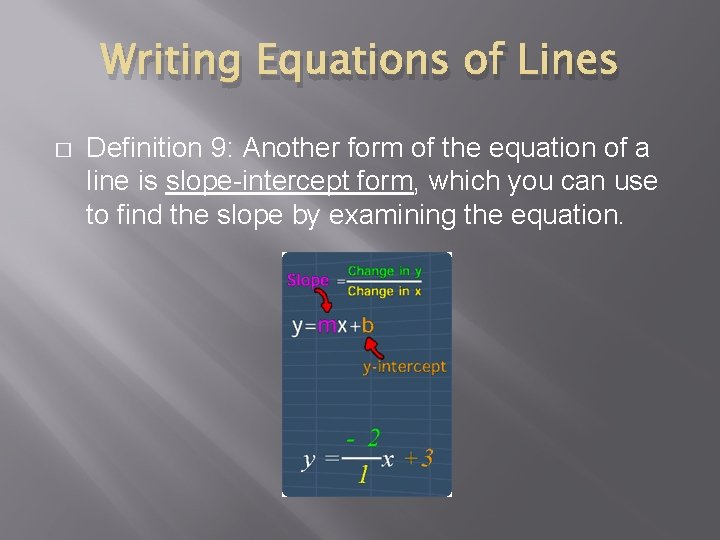Writing Equations of Lines � Definition 9: Another form of the equation of a line is slope-intercept form, which you can use to find the slope by examining the equation.Examples 16 – 19 � 16. Find the slope of each line. 4 x + 3 y = 7 17. 3 x + 2 y = 1 18. 2/3 x + 1/2 y = 1 19. Ax + By = C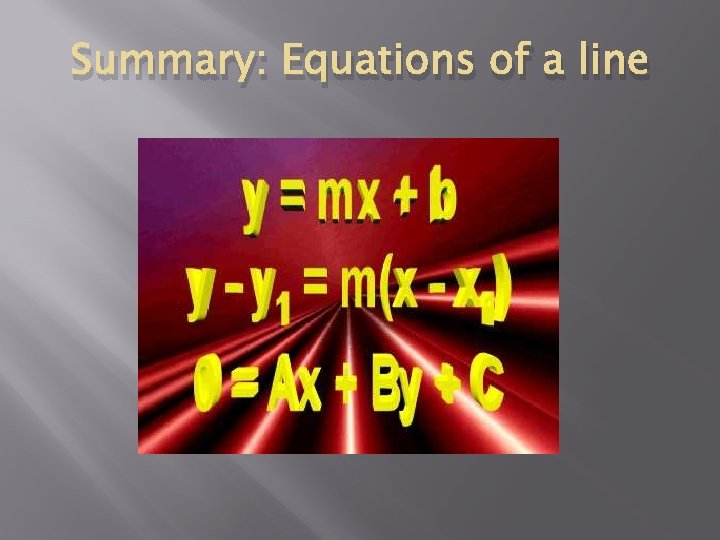Summary: Equations of a lineTOTD � Write in point-slope form the equation of the line through each pair of points. � (-10, 3) and (-2, -5)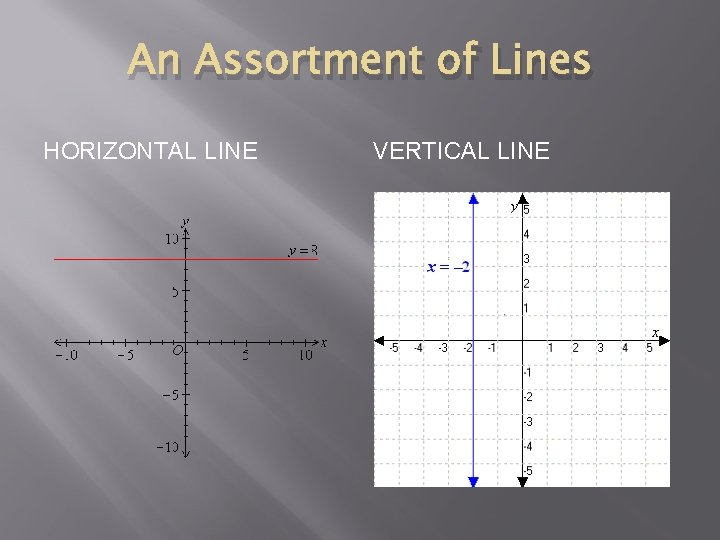An Assortment of Lines HORIZONTAL LINE VERTICAL LINE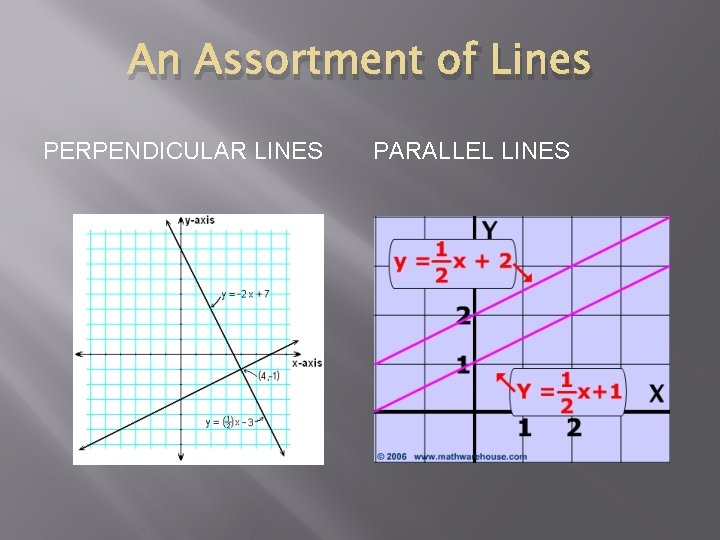An Assortment of Lines PERPENDICULAR LINES PARALLEL LINESExamples 20 – 21 � 20. 21. Find the given lines. Find the equation of a line that is perpendicular to y = 3/4 x + 2 and passes through the point (0, 4). Find the equation that goes through the point (-1, 3) and is perpendicular to the line y = 5 x – 3.Examples 22 – 23 Find the given lines. 22. Find the equation of a line that is parallel to y = 2/3 x + 5/8 and passes through the point (2, 1). � 23. Find the equation that is vertical and passes through the point (5, -3)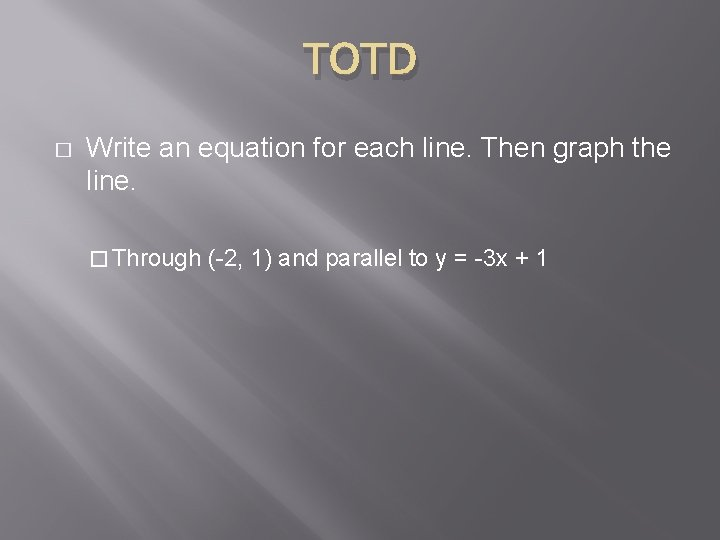TOTD � Write an equation for each line. Then graph the line. � Through (-2, 1) and parallel to y = -3 x + 1